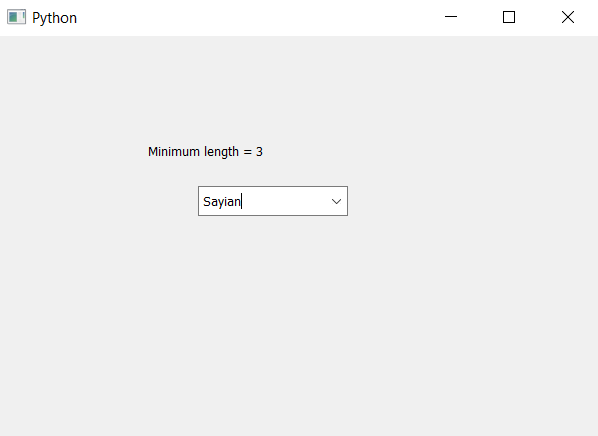# PyQt5 – Getting minimum content length to Combo Box item | minimumContentsLength() method

• Last Updated : 11 Nov, 2021

In this article we will see how we can get the minimum length to the items of combo box i.e this property holds the minimum number of characters that should fit into the combo box. In order to do this we will use setMinimumContentsLength method, by default minimum length of combo box is 0.
In order to get the minimum content length we will use minimumContentsLength method.

Syntax : combo_box.minimumContentsLength()
Argument : It takes no argument
Return : It returns integer

Below is the implementation –

## Python3

 `# importing libraries``from` `PyQt5.QtWidgets ``import` `*``from` `PyQt5 ``import` `QtCore, QtGui``from` `PyQt5.QtGui ``import` `*``from` `PyQt5.QtCore ``import` `*``import` `sys`  `class` `Window(QMainWindow):` `    ``def` `__init__(``self``):``        ``super``().__init__()` `        ``# setting title``        ``self``.setWindowTitle(``"Python "``)` `        ``# setting geometry``        ``self``.setGeometry(``100``, ``100``, ``600``, ``400``)` `        ``# calling method``        ``self``.UiComponents()` `        ``# showing all the widgets``        ``self``.show()` `    ``# method for widgets``    ``def` `UiComponents(``self``):` `        ``# creating a combo box widget``        ``self``.combo_box ``=` `QComboBox(``self``)` `        ``# setting geometry of combo box``        ``self``.combo_box.setGeometry(``200``, ``150``, ``150``, ``30``)` `        ``# geek list``        ``geek_list ``=` `[``"Sayian"``, ``"Super Saiyan"``, ``"Super Sayian 2"``,``                                              ``"Super Sayian B"``]` `        ``# making it editable``        ``self``.combo_box.setEditable(``True``)` `        ``# adding list of items to combo box``        ``self``.combo_box.addItems(geek_list)` `        ``# setting minimum content length``        ``self``.combo_box.setMinimumContentsLength(``3``)` `        ``# getting minimum content length``        ``mini ``=` `self``.combo_box.minimumContentsLength()` `        ``# creating label to show minimum length``        ``label ``=` `QLabel(``"Minimum length = "` `+` `str``(mini), ``self``)` `        ``# setting geometry of the label``        ``label.setGeometry(``150``, ``100``, ``350``, ``30``)`  `# create pyqt5 app``App ``=` `QApplication(sys.argv)` `# create the instance of our Window``window ``=` `Window()` `# start the app``sys.exit(App.``exec``())`

Output :My Personal Notes arrow_drop_up